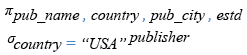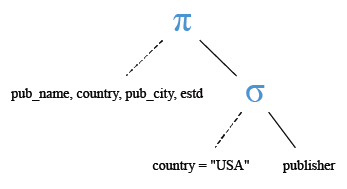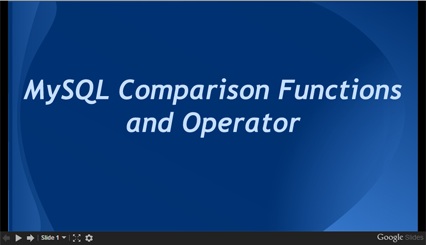# MySQL equal operator

## equal operator

MySQL equal operator performs an equality comparison.

Syntax:

`=`

MySQL Version: 5.6

Example: MySQL equal operator

The following MySQL statement checks if 1 is equal to 1, if 1 is equal to 2, if NULL is equal to NULL, if NULL is equal to 3 and if 3 is equal to NULL.

Code:

``````SELECT 1 = 1, 1=2,NULL = NULL, NULL=3,3= NULL;
```
```

Sample Output:

```mysql> SELECT 1 = 1, 1=2,NULL = NULL, NULL=3,3= NULL;
+-------+-----+-------------+--------+---------+
| 1 = 1 | 1=2 | NULL = NULL | NULL=3 | 3= NULL |
+-------+-----+-------------+--------+---------+
|     1 |   0 |        NULL |   NULL |    NULL |
+-------+-----+-------------+--------+---------+
1 row in set (0.00 sec)
```

Example: MySQL equal operator with WHERE clause

The following MySQL statement will fetch the rows after checking whether the publisher belongs to the USA.

Code:

``````SELECT pub_name,country,pub_city,estd
FROM publisher
WHERE country='USA';
```
```

Relational Algebra Expression:Relational Algebra Tree:Sample table: publisher

Sample Output:

```mysql> SELECT pub_name,country,pub_city,estd
-> FROM publisher
-> WHERE country='USA';
+--------------------------+---------+----------+------------+
| pub_name                 | country | pub_city | estd       |
+--------------------------+---------+----------+------------+
| Jex Max Publication      | USA     | New York | 1969-12-25 |
| Mountain Publication     | USA     | Houstan  | 1975-01-01 |
| Summer Night Publication | USA     | New York | 1990-12-10 |
+--------------------------+---------+----------+------------+
3 rows in set (0.00 sec)
```

PHP script:

``````<!doctype html>
<html lang="en">
<meta charset="utf-8">
<meta name="viewport" content="width=device-width, initial-scale=1">
<title>example-equal-operator- php mysql examples | w3resource</title>
<meta name="description" content="example-equal-operator- php mysql examples | w3resource">
<body>
<div class="container">
<div class="row">
<div class="col-md-12">
<h2>A list of the publishers who belog to USA. List also includes their date of country,city, date of establishment:</h2>
<table class='table table-bordered'>
<tr>
<th>Publishers</th><th>Country</th><th>City</th><th>Date of establishment</th>
</tr>
<?php
\$hostname="your_hostname";
\$db = "your_dbname";
foreach(\$dbh->query('SELECT pub_name,country,pub_city,estd
FROM publisher
WHERE country="USA"') as \$row) {
echo "<tr>";
echo "<td>" . \$row['pub_name'] . "</td>";
echo "<td>" . \$row['country'] . "</td>";
echo "<td>" . \$row['pub_city'] . "</td>";
echo "<td>" . \$row['estd'] . "</td>";
echo "</tr>";
}
?>
</tbody></table>
</div>
</div>
</div>
</body>
</html>
```
```

View the example in browser

JSP script:

``````<%@page contentType="text/html" pageEncoding="UTF-8"%>
<%@ page import="java.sql.*" %>
<%@ page import="java.io.*" %>
<!DOCTYPE html>
<html>
<meta http-equiv="Content-Type" content="text/html; charset=UTF-8">
<title>example-equal-operator</title>
<body>
<%
try {
Class.forName("com.mysql.jdbc.Driver").newInstance();
String Host = "jdbc:mysql://localhost:3306/w3resour_bookinfo";
Connection connection = null;
Statement statement = null;
ResultSet rs = null;
connection = DriverManager.getConnection(Host, "root", "datasoft123");
statement = connection.createStatement();
String Data ="SELECT pub_name,country,pub_city,estd FROM publisher WHERE country='USA'";
rs = statement.executeQuery(Data);
%>
<TABLE border="1">
<tr width="10" bgcolor="#9979">
<td>Publisher</td>
<td>Country</td>
<td>City</td>
<td>Date of establishment</td>
</tr>
<%
while (rs.next()) {
%>
<TR>
<TD><%=rs.getString("pub_name")%></TD>
<TD><%=rs.getString("country")%></TD>
<TD><%=rs.getString("pub_city")%></TD>
<TD><%=rs.getString("estd")%></TD>
</TR>
<%   }    %>
</table>
<%
rs.close();
statement.close();
connection.close();
} catch (Exception ex) {
out.println("Can’t connect to database.");
}
%>
</body>
</html>
```
```

Online Practice Editor:

Slideshow of MySQL Comparison Function and Operators﻿